# HOMER Grid 1.4

 Navigation: HOMER's Calculations Operation of a Cofired GeneratorA cofired generator operates on a mixture of fossil fuel and biogas. In each time step, HOMER calculates the required output of the generator and the corresponding mass flow rates of fossil fuel and biogas. This calculation is based on several key assumptions:

1.The biogas substitution ratio (zgas) is a constant, independent of engine output power or fuel mixture.

2.The system always attempts to maximize the use of biogas and minimize the use of fossil fuel.

3.The fossil fraction cannot go below a certain minimum.

4.Even if the derating factor associated with operating in dual-fuel mode is less than 100%, the generator can produce up to 100% of its rated power, provided the fossil fraction is high enough.

The fuel curve of a cofired generator defines the fuel consumption of the generator in pure fossil mode. So, the fossil fuel consumption in pure fossil mode is given by the following equation. (See the table of nomenclature below for a definition of all the symbols.)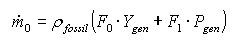(1)

And from assumption 1: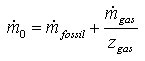∴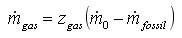(2)

Where zgas is the biogas substitution ratio. Now from the definition of the fossil fraction: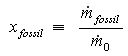(3)

Using equations 2 and 3:∴(4)

But for a given value of Pbio, the value of xfossil is unknown so the above equation is not enough on its own to solve for the biogas flow rate. From assumption 2, we want to maximize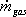, which means we want to minimize xfossil. But from assumption 3:where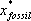is the minimum fossil fraction required for ignition. So the target value forcorresponds to . Using equation 4: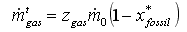(5)

But there are two independent upper limits on the actual value of. At the minimum fossil fraction, the output of the generator is limited to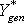, defined as follows: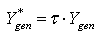where τ, the derating factor, is less than or equal to 1. This limitation can be implemented by imposing an upper limit oncorresponding toand. Using equations 1 and 4, this maximum value can be defined as: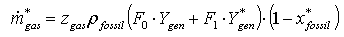(6)

This upper limit can be thought of as a physical limitation—the maximum rate at which biogas can be ingested in the engine. The available biomass resource, agas, constitutes the other upper limit on. So the actual value ofis the minimum of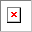,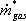, and agas: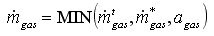(7)

Knowing the value of, we can determine xfossil. Solving equation 4 for xfossil: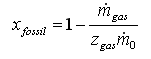(8)

And from equation 3: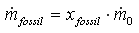(9)

So at any time step, given particular values of Pbio and agas, the biogas flow rate and the fossil fuel flow rate can be calculated from equations 7 and 9, respectively.

Table of Nomenclature

 Symbol Units Description ρfossil kg/L Density of fossil fuel τ % Generator derating factor agas kg/hr Available biogas flow rate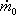kg/hr Fossil fuel flow rate (in pure fossil mode)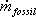kg/hr Fossil fuel flow rate (in dual-fuel mode)kg/hr Biogas flow rate (in dual-fuel mode)kg/hr Maximum value of biogas flow rate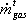kg/hr Target value of biogas flow rate xfossil % Fossil fraction% Minimum fossil fraction zgas none Biogas substitution ratio F0 L/hr/kW Generator fuel curve intercept coefficient F1 L/hr/kW Generator fuel curve slope Pgen kW Power output of the generatorkW Maximum output of generator at minimum fossil fraction Ygen kW Rated capacity of the generator

The Homer Support site has a searchable knowledgebase and additional support options. HOMER Online contains the latest information on model updates, as well as sample files, resource data, and contact information. ©2017 HOMER Energy, LLC